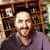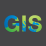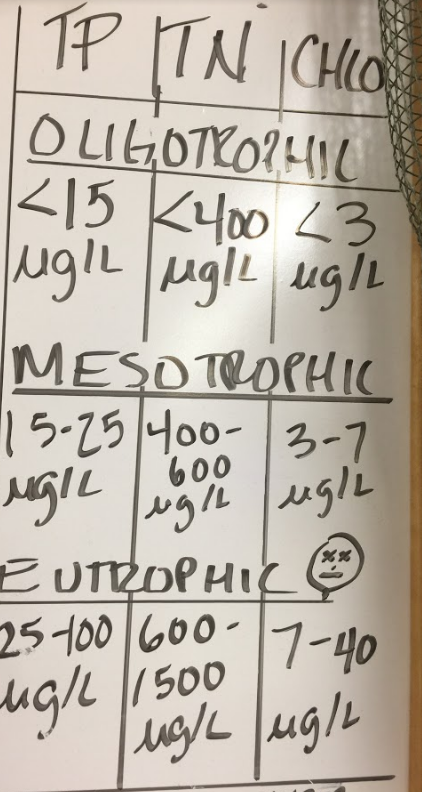# If statement calculation, Survey123

27071
16
03-05-2018 10:57 AMNew Contributor II

I am working on creating form with multiple repeats/questions.  What I am trying to do is calculate an answer to a question based on the which answer was chosen from the previous select_one question.  I will use sample data for simplicity sake.

select_one color: green, blue, red, yellow

calculation results based on color chosen (what I am trying): if(selected(\${color}, 'red', 'Cardinal', if(selected(\${color}, 'green', 'Parrot', if(selected(\${color}, 'blue', 'Bluebird', if(selected(\${color}, 'yellow', 'Finich', 'N/A'))))))))

I have received multiple different errors for trying this multiple ways, the current error is "requires 3 arguments, only provided 1"...Sometimes I get "requires 3 arguments, only provided 4 or 5 etc"

What am I doing incorrectly?

Tags (2)
16 RepliesNew Contributor II

I figured it out, took the "selected" out and eventually got everything correct.byEsri Notable Contributor

Hi Mary,

Glad you got it working. The reason your calculation was not working is that you are missing closing brackets on your selected statement. It should be similar to: selected(\${question},'answer').

Therefore using a selected statement in an if statement: if(selected(\${question},'answer'),'true','false')

And you can then nest the if statements as you have done in your original calculation.

You can find some good info in this blog: https://community.esri.com/groups/survey123/blog/2016/05/28/the-art-of-hiding

Phil.Occasional Contributor

Hi All, I believe I'm attempting to do the same thing however I'm fighting the syntax and was hoping for assistance.

Essentially a pre selected culvert type determines the expression used to calculate a value. So it I select culvert type A do this math, if I select culvert type B do different math ect with culvert types C,D,E,F

So far I have (if culvert type CSP is selected the math is just the IS rating * 2.

if((selected(\${CulvertType},'C.S.P'),int(\${InvertSedimentRating}*2),0))

But I'm struggling to get more values in for the selected (the below line doesn't work can't figure out why)

if(selected(\${CulvertType},'C.S.P'),int(\${InvertSedimentRating}*2),0),if(selected(\${CulvertType},'H.D.P.E'),int(\${InvertSedimentRating}*3.2),0)Occasional Contributor II

The way the statement is written, your first else case is 0, when it needs to be the next if() statement. Basically, it reads as:

if culvert type is CSP

then int(\${InvertSedimentRating}*2

else 0

----- the function stops here, because that is a full statement. Anything else after this in the same calculation either isn't evaluated or just plain breaks.

In order to nest your if() statements, the next if() needs to take the place of the preceding if()'s else case. Simply remove the "0)," from the first if() in your calculation, and close up the parentheses at the end:

if(selected(\${CulvertType},'C.S.P'),int(\${InvertSedimentRating}*2),if(selected(\${CulvertType},'H.D.P.E'),int(\${InvertSedimentRating}*3.2),0))

if culvert type is CSP

then int(\${InvertSedimentRating}*2

else if culvert type is HDPE

then int(\${InvertSedimentRating}*3.2

else 0Occasional Contributor

Perfect thanks Nick!Occasional Contributor

Hi,

I included an integer type question for getting the number of truck axles (truck_axles: from 2 to 8), and a decimal type question for getting the truck load percentage(load_pct). Each truck axle value has a theoretical load capacity (theo_load_weight). In my calculation to get the effective weight (load_weight), I must match the theoretical load capacity, from the axles number, and then multiply by the load percentage

Not sure if I should use an IF

if(selected(\${truck_axles},'2'),...?byEsri Esteemed Contributor

Hi Rene,

An if() statement sequence may work for what you are doing. An alternative would be to have the theo_load_weight data in a CSV and use pulldata() to look up the value based on the number of axles.  See Formulas—Survey123 for ArcGIS | ArcGIS for more information on pulldata().Occasional Contributor

Hello, I have 4 types of truck monitoring (Type_Suivi): ImportationREM, Reutilisation, Elimination and Entreposage. For question WBS_Origine, i set a relevant condition where truck monitoring is ImportationREM, but then for that same question, i use this statement in the calculation cell.:

if (selected(\${Type_Suivi},'Elimination'),\${WBS_id},if(selected(\${Type_Suivi},'Reutilisation'),\${WBS_id},if (selected(\${Type_Suivi},'Entreposage'),\${WBS_id},’0’)))

Doing so, all questions after that one are omitted. Is the relevant condition blocking the calculation and everthing else that follows?

WBS_id is a text variable for storing coded areas of the project site that am attempting to write to the WBS_Origine

ThanksNew Contributor II

Hello, I have a survey for collecting water data, and want to symbolize the map based off of collected values. I figured using a calculation field in the survey would be a good work around, as I could just based the symbology off that field. Basically I need to know if the water is "Oligotrophic", "Mesotrophic", or "Eutrophic" based on the following values:Can anybody provide any suggestions. I'm pretty new to Survey123, and the calculation I am trying to use is long and likely erroneous:

if(\${P}<15,and \${TN}<400,and\${ChloroA}<3),"Oligotrophic"), if(\${P}>15 and <=25, and\${TN}>400 and <=600,and\${ChloroA}>3 and <=600),"Mesotrophic"), if(\${P}>25,and \${TN}>=600,and\${ChloroA}>=7),"Eutrophic")

Thanks in advanced for any help!